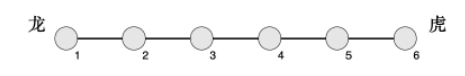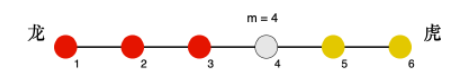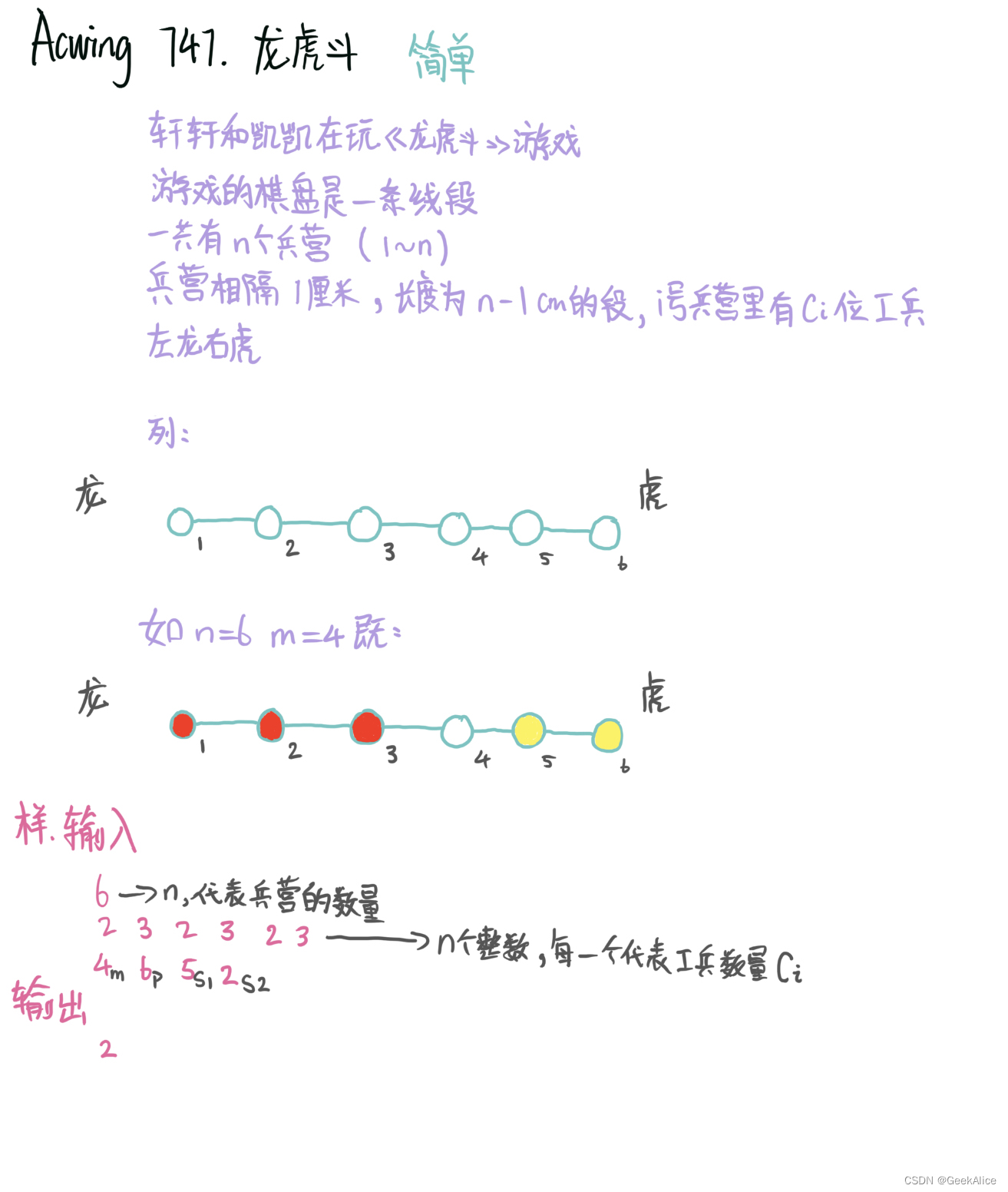# AcWing 474. 龙虎斗1<m<n
1≤p1≤n
n≤105,ci,s1,s2≤109

``````6
2 3 2 3 2 3
4 6 5 2
``````

``2````````#include<bits/stdc++.h>
using namespace std;
long long m,p1,s1,s2,fi;
int n;
int main()
{
long long MIN=1e19,w;
long long su1=0,su2=0;
long long k1,k2;
scanf("%d",&n);
for(int i=1;i<=n;i++)
scanf("%lld",&fi[i]);
scanf("%lld %lld %lld %lld",&m,&p1,&s1,&s2);
fi[p1]+=s1;
for(int i=1;i<=n;i++){
if(i<m)
su1+=(m-i)*fi[i];
else if(i>m)
su2+=(i-m)*fi[i];
}
for(int i=1;i<=n;i++){
k1=su1;
k2=su2;
if(i<m)
k1+=(m-i)*s2;
else if(i>m)
k2+=(i-m)*s2;
long long tep=abs(k1-k2);
if(tep<MIN){
MIN=tep;
w=i;
}
}
printf("%lld",w);
return 0;
}
``````